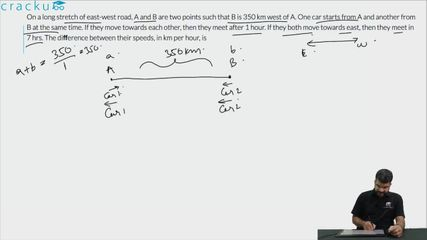Question 99

# On a long stretch of east-west road, A and B are two points such that B is 350 km west of A. One car starts from A and another from B at the same time. If they move towards each other, then they meet after 1 hour. If they both move towards east, then they meet in 7 hrs. The diﬀerence between their speeds, in km per hour, is

Solution

Let 'a' and 'b' be the speed (in km/hr) of cars starting from both A and B respectively.

If they both move in east direction, then B will catch A if and only if b > a.

Relative speed of both the cars when they move in east direction = (b - a) km/hr

It takes them 7 hours to meet. i.e. they travel 350 km in 7 hours with a relative speed of (b-a) km/hr.

Hence, (b - a) = $$\dfrac{350}{7}$$ = 50 km/hr.

### View Video Solution• All Quant CAT Formulas and shortcuts PDF
• 30+ CAT previous papers with solutions PDF